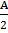# A practice is executing simple harmonic motion (SHM) of amplitude A, along the x-axis, about x=0.  When is potential Energy (PE) equals kinetic energy (KE), the position of the particle will be a.b.c.d. A

## Question ID - 50118 :- A practice is executing simple harmonic motion (SHM) of amplitude A, along the x-axis, about x=0.  When is potential Energy (PE) equals kinetic energy (KE), the position of the particle will be a.b.c.d. A

3537

Potential Energy (U) =Kinetic energy (K) =According to the question, U= k= -x = ±Next Question :

A musician suing an open flute of length 50 cm produces second harmonic sound waves.  A persons runs towards the musician from another end of a hall at a speed of 10km/h.  If the wave speed is 330 m/s, the frequency heard by the running person shall be close to

 a. 753 Hz b. 500 Hz 333 Hz d. 666 Hz